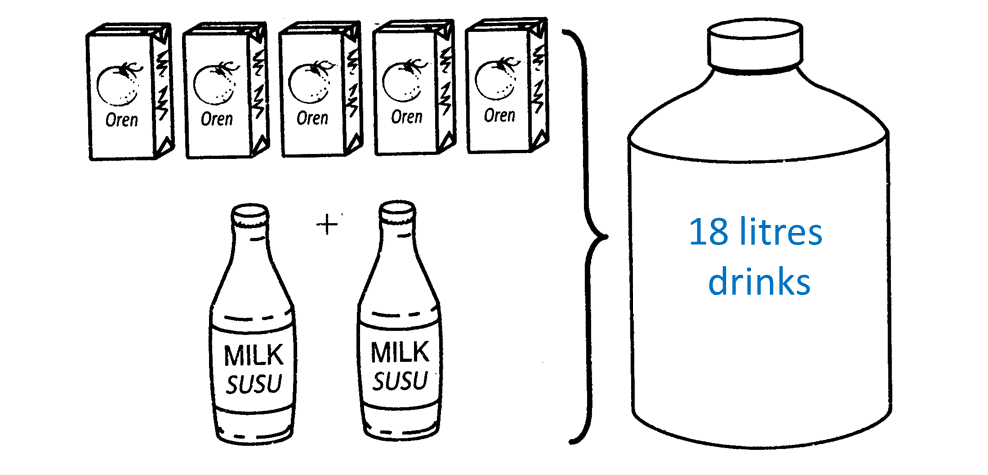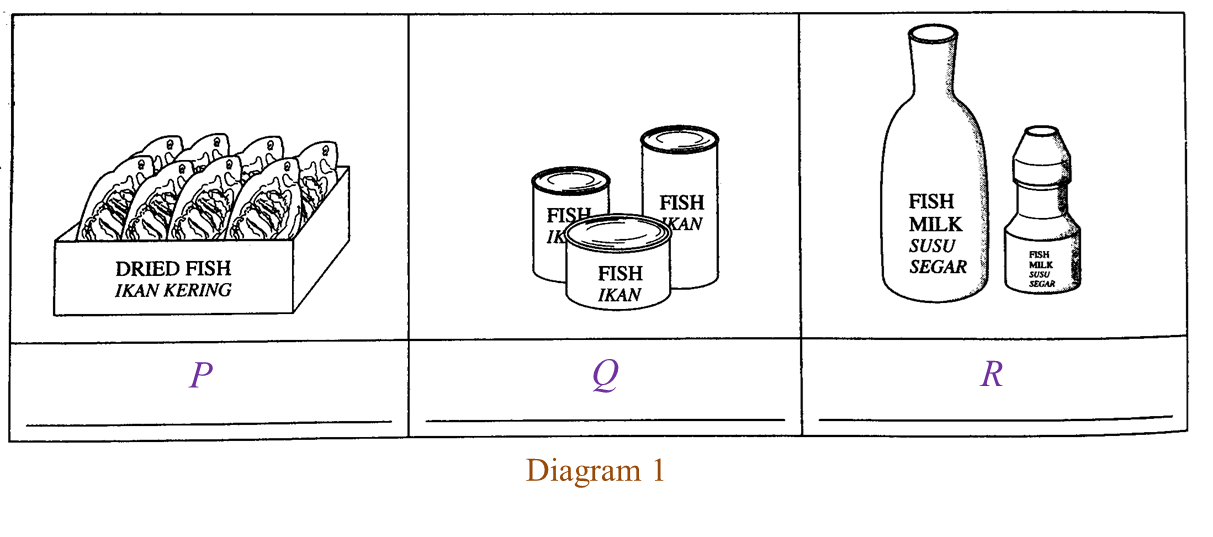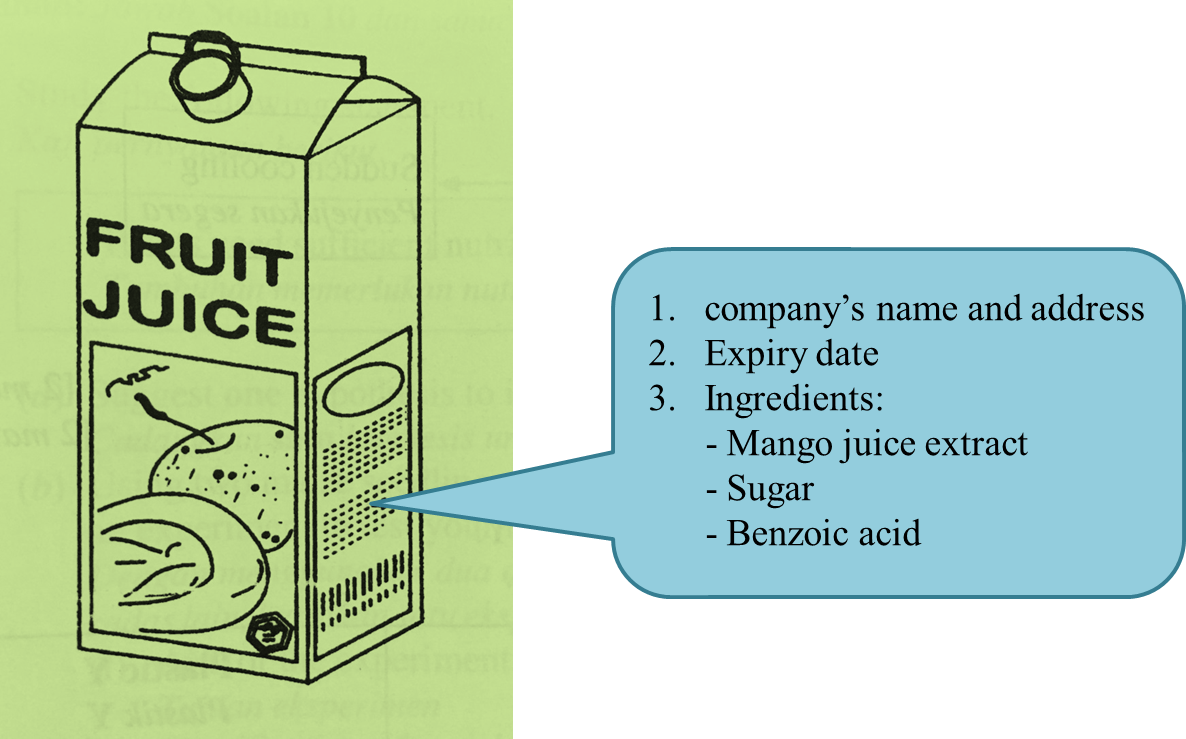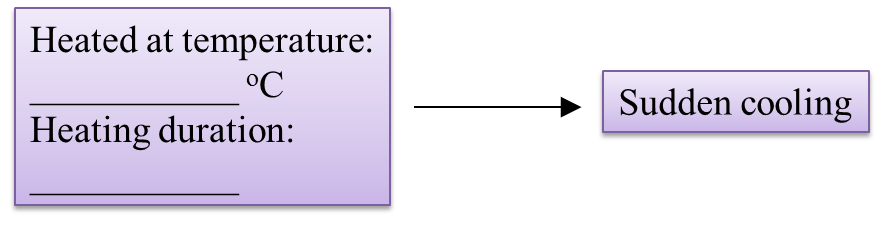# 12.2.2 Linear Inequalities, PT3 Focus Practice

Question 6:
List all the integer values of x which satisfy the following linear inequalities:
–2 < 3x + 1 ≤ 10

Solution:
–2 < 3x + 1
–3 < 3x
x > –1
x = 0, 1, 2, 3, …

3x + 1 ≤ 10
3x ≤ 9
x ≤ 3
x = 3, 2, 1, 0, …

Therefore x = 0, 1, 2, 3

Question 7:
List all the integer values of x which satisfy the following linear inequalities:
–5 < 2x – 3 ≤ 1

Solution:
–5 < 2x – 3
–5 + 3 < 2x
2x > –2
x > –1
x = 0, 1, 2, 3, …

2x – 3 ≤ 1
2x ≤ 4
x ≤ 2
x = 2, 1, 0, –1, …

Therefore x = 0, 1, 2

Question 8:

Solution:

Question 9:
Find the biggest and the smallest integer of x that satisfy
3x + 2 ≥ –4 and 4 – x > 0.

Solution:
3x + 2 ≥ –4
3x ≥ –4 – 2
3x ≥ –6
x ≥ –2

4 – x > 0
x > –4
x < 4

Smallest integer of x is –2, and the biggest integer of x is 3.

Question 10:

Solution:

$\begin{array}{l}7-\frac{h}{2}\le 5\\ -\frac{h}{2}\le 5-7\\ -h\le -4\\ h\ge 4\\ \\ 3\left(h+2\right)\le 20+h\\ 3h+6\le 20+h\\ 2h\le 14\\ h\le 7\\ \\ 4\le h\le 7\\ \therefore x=4,y=7\end{array}$

# 12.2.1 Linear Inequalities, PT3 Focus Practice

                 
         
12.2.1 Linear Inequalities, PT3 Focus Practice
          

         Question 1:
          
Draw a number line to represent the solution for the linear inequalities –3 < 5 – x  ≤ 4.
          

          
Solution:
          
–3 < 5 – x   and   5 – x  ≤ 4
          
x < 5 + 3   and   x  ≤ 4 – 5
          
x < 8   and   x ≤ –1 → x  ≥ 1
         
          

          Thus, the solution is 1 ≤ x < 8
          
          
             
  
                 
         
Question 2:
          
Solve the following simultaneous linear inequalities.
          
                       
          
          

          Solution:
          
3x – 5 ≤ 1
          
3x  ≤ 1 + 5
          
3x  ≤ 6
          
x  ≤ 2

          
          
          
                    
The solution is –3 < x  ≤ 2.
       
       
  
  
                 

         
Question 3:
          
The solution for the inequality 2 + < 3x – 4 is
          

          
Solution:
          
2 + x < 3x – 4
          
x – 3x < –4 – 2
          
–2x < –6
          
x < –3
          
x > 3
       
       
  
  
                 
         
Question 4:
          
The solution for the inequality –2 (6y + 3) < 3 (4 – 2y) is
          

         
          
Solution:
          
–2 (6y + 3) < 3 (4 – 2y)
          
–12y – 6 < 12 – 6y
          
–12y + 6< 12 + 6
          
–6y < 18
          
y < 3
          
y > –3
       
       
  
  
                 
         
Question 5:
          
Solve each of the following inequalities.
          
(a) 3x + 4 > 5x – 10
          
(b)   –3  ≤ 2x + 1 < 7
          

         
          
Solution:
          
(a)
          
3x + 4 > 5x – 10
          
3x – 5x > –10 – 4
          
–2x > –14
          
x > –7
          
x < 7
       
          
          

          (b)
          
–3 ≤ 2x + 1 < 7
          
–3 ≤ 2x + 1   and   2x + 1 < 7
          
–2x  ≤ 1 + 3   and   2x < 7 – 1
          
–2x  ≤ 4 and   2x < 6
          
≥ –2   and   x < 3
  
                 
The solution is –2 ≤ x < 3.
       
       
  

# 12.1 Linear Inequalities

12.1 Linear Inequalities

12.1.1 Inequalities
1.   To write the relationship between two quantities which are not equal, we use the following inequality signs:
> greater than
< less than
≥ greater than or equal to
≤ less than or equal to

2.   7 > 4 also means 4 < 7.  7 > 4 and 4 < 7 are equivalent inequalities.

12.1.2  Linear Inequalities in One Unknown
1.   An inequality in one unknown to the power of 1 is called a linear inequality.
Example: 2x + 5 > 7

2.
A linear inequality can be represented on a number line.

12.1.3 Computation on Inequalities
1.   When a number is added or subtracted from both sides of an inequality, the condition of the inequality is unchanged.
Example:
Given 5 > 3
Then, 5 + 2 > 3 + 2 ← (symbol ‘>’ remains)
Hence, 7 > 5

2.   When both sides of an inequality are multiplied or divided by the same positive number, the condition of the inequality is unchanged.
Example:
Given 4x ≤ 16
Then, 4x ÷ 4 ≤ 16 ÷ 4 ← (symbol ‘≤’ remains)
Hence, x ≤ 4

3.   When both sides of an inequality are multiplied or divided by the same negative number, the inequality is reversed.
Example:
Given –3 > –5
Hence, 3 < 5
Given –5y > –10
Then, –5y ÷ 5 > –10 ÷ 5
–y > –2
Hence, y < 2

12.1.4 Solve Inequalities in One Variable
To solve linear inequalities in one variable, use inverse operation to make the variable as the subject of the inequality.

Example:
Solve the following linear inequalities.

Solution:
(a)

(b)

12.1.5 Simultaneous Linear Inequalities in One Variable
1.   The common values of two simultaneous inequalities are values which satisfy both linear inequalities.
The common values of the simultaneous linear inequalities x ≤ 3 and x > –1 is –1 < x ≤ 3.

2.   To solve two simultaneous linear inequalities is to find a single equivalent inequality which satisfies both inequalities.

# 6.3.2 Practising Critical and Analytical Thinking when Selecting Processed Food (Structured Questions)

Question 1:
Diagram 1 shows a labelled container of a processed food.(a) Based on Diagram 1, identify two chemicals written on the label. [2 marks]

(b) Based on the label in Diagram 1, which chemical is used as a preservative? [1 mark]

(c) Name the method of food processing used in Diagram 1. [1 mark]

(d) What other information should be written on the label in Diagram 1 according to Food Regulations 1985?

(a)
1. Benzoic acid
2. Flavouring

(b)

Benzoic acid

(c)

Canning

(d)

- Name of manufacturer

# 11.2.2 Linear Equations II, PT3 Focus Practice

 
Question 6:
Diagram below shows 5 boxes of orange juice and 2 bottles of milk. Both orange juice and milk are mixed to produce 18 litres drink.(a) Based on the above situation, write a linear equation.
(b) If 9 litres of drinks is produced by using 2 boxes of orange juice and 2 bottles of milk, find the volume, in litre, of orange juice in each box.

Solution:
Let j be the number of boxes of orange juice and m be the number of bottles of milk.
(a)
5j + 2m = 18

(b)

 
 

# 11.2.1 Linear Equations II, PT3 Focus Practice

11.2.1 Linear Equations II, PT3 Focus Practice

Question 1:
It is given that 2x = 6 and 3x + y = 10.
Calculate the value of y.

Solution:
2x = 6
= 3
Substitute = 3 into 3x + y = 10
3 (3) + y = 10
= 10 – 9
y = 1

Question 2:
It is given that = –1 and x – 3y = –10.
Calculate the value of x.

Solution:
Substitute = –1 into x – 3y = –10
– 3 (–1) = –10
+ 3 = –10
= –10 – 3
x = –13

Question 3:
It is given that 7x – 2y = 19 and x + = 13.
Calculate the value of y.

Solution:
Using Substitution method
7x – 2y = 19 -------- (1)
+ y = 13 ------- (2)
From equation (2),
x = 13 – y ------- (3)
Substitute equation (3) into equation (1),
7x – 2y = 19
7 (13 – y) – 2y = 19
91 – 7y – 2y = 19
– 9y = 19 – 91
– 9y = –72
y = 8

Question 4:
It is given that 2xy = 5 and 3x – 2= 8.
Calculate the value of x.

Solution:
Using Elimination method
2xy = 5 -------- (1)
3– 2y = 8 ------- (2)
(1) × 2:  4– 2y = 10 -------- (3)
3x – 2= 8 ------- (2)
(3) – (2): x – 0 = 2
x = 2

Question 5:
It is given that + 2y = 4 and x + 6y = –4.
Calculate the value of x.

Solution:
Using Elimination method
+ 2y = 4 -------- (1)
+ 6y = –4 ------- (2)
(1) × 3:  3x + 6y = 12 -------- (3)
x + 6y = –4 ------- (2)
(3) – (2): 2= 12 – (–4)
2x = 16
x = 8

# 6.3.1 The Methods and Substances Used in Food Technology (Structured Questions)

Question 1:
Diagram 1 shows foods that have been processed through three different methods.(a) Write down the food processing methods, P, Q and R, in Diagram 1. [3 marks]

(b) What happens to the water content in the food that has been processed through method P? [1 mark]

(c) The food is heated at 121 oC during the process in method Q.
What is the purpose of the heating? [1 mark]

(d) Fresh milk is heated for 30 minutes in method R.
What is the temperature used? [1 mark]

(a)
P: Dehydration
Q: Canning
R: Pasteurisation

(b)
Water is removed from the food.

(c)
To kill the microorganisms in the food.

(d)
63 oC

Question 2:
Diagram 2.1 shows a food label of fruit juice that has been processed through a method of food processing according to the Food Regulations 1985.Diagram 2.1

(a) Name the preservative added into the fruit juice in Diagram 2.1. [1 mark]

(b) What information is not shown on the food label in Diagram 2.1 as required by the Food Regulations 1985? [1 mark]

(c) Name the food processing method in Diagram 2.1. [1 mark]

(d) Besides fruit juice, state another drink that can be processed by the method in 2(c). [1 mark]

(e) Based on Diagram 2.2, state the temperature and heating duration during the food processing method in 2(c). [1 mark]Diagram 2.2

(a) Benzoic acid.

(b)
Net weight / Volume of liquid.

(c)
Pasteurization

(d) Milk

(e)
Temperature: 63 oC
Heating duration: 30 minutes
OR
Temperature: 72 oC
Heating duration: 15 seconds

# 11.1 Linear Equations II

11.1 Linear Equations II

11.1.1 Linear Equations in Two Variables
1.   A linear equation in two variables is an equation which contains only linear terms and involves two variables.

2.
If the value of one variable in an equation is known, then the value of the other variable can be determined and vice versa.
Example:
Given that 2x + 3y = 6, find the value of
(a) x when y = 4, (b) y when x = –3

Solution:
(a) Substitute y = 4 into the equation.
2x + 3y = 6
2x + 3 (4) = 6
2x + 12 = 6
2= 6 – 12
2= –6
x = –3

(b)   Substitute x = –3 into the equation.
2x + 3y = 6
2 (–3) + 3y = 6
–6 + 3y = 6
3= 6 + 6
3= 12
y = 4

3.   A linear equation in two variables has many possible solutions.

11.1.2  Simultaneous Linear Equations in Two Variables
1.  Two equations are said to be simultaneous linear equations in two variables if
(a) Both are linear equations in two variables, and
(b) Both involve the same variables.
Example: 2x + y = 9, x = 2+ 1

2.   The solution of two simultaneous linear equations in two variables is any pair of values (x, y) that satisfied both the equations.
3.   Simultaneous linear equations in two variables can be solved by the substitution method or the elimination method.

Example:
Solve the following simultaneous linear equation.
2x + y = 9
3xy = –4

Solution:
(A)  Substitution method
2x + y = 9 -------- (1) label the equations as (1) and (2)
3xy = –4 ------- (2)

From equation (1),
y = 9 – 2x ------- (3) expressing y in terms of x.
Substitute equation (3) into equation (2),
3x – (9 – 2x) = –4
3x – 9 + 2x = –4
5x = –4 + 9
5x = 5
x = 1

Substitute = 1 into equation (1),
2 (1) + y = 9
2 + y = 9
y = 9 – 2
y = 7
The solution is x = 1, y = 7.

(B) Elimination method
2x + y = 9 -------- (1) ← Both equations have the same coefficient of y.
3y = –4 ------- (2)
(1) + (2): 2x + 3x = 9 + (–4) y + (–y) = 0
5x = 5
x = 1

Substitute = 1 into equation (1) or (2),
2x + y = 9 -------- (1)
2 (1) + y = 9
y = 9 – 2
y = 7

The solution is x = 1, y = 7.